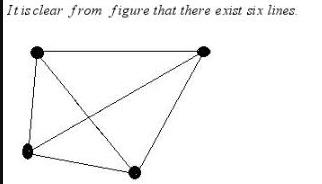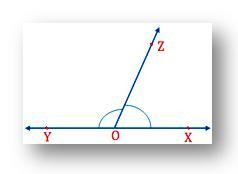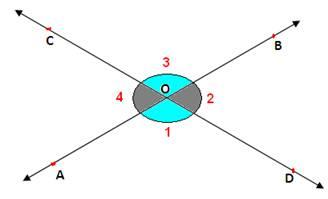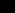# Test: Basic Concepts Of Geometry

## 10 Questions MCQ Test Mathematics (Maths) Class 9 | Test: Basic Concepts Of Geometry

Description
This mock test of Test: Basic Concepts Of Geometry for Class 9 helps you for every Class 9 entrance exam. This contains 10 Multiple Choice Questions for Class 9 Test: Basic Concepts Of Geometry (mcq) to study with solutions a complete question bank. The solved questions answers in this Test: Basic Concepts Of Geometry quiz give you a good mix of easy questions and tough questions. Class 9 students definitely take this Test: Basic Concepts Of Geometry exercise for a better result in the exam. You can find other Test: Basic Concepts Of Geometry extra questions, long questions & short questions for Class 9 on EduRev as well by searching above.
QUESTION: 1

### The measure of an angle which is a supplement of itself is :

Solution:

Let the angle = x
Supplement of angle= 180-x

ATQ

180-x = x
180 = 2x

x = 90°

And its supplement will be 180-90=90°

QUESTION: 2

### Two lines are parallel to each other, only when

Solution:

Two lines in a plane are said to be parallel if they do not intersect, when extended infinitely in both the direction.

Also, the distance between the two lines is the same throughout.

QUESTION: 3

### The angle whose complement is one-third of its supplement will be

Solution:

Let the angle be x
complement of x is (90 − x)°
supplement of x is (180 − x)°
Given (90 − x)° = (1/3) × (180 − x)°
⇒ 270° − 3x = 180°− x
⇒ 270° − 180° = 3x − x
⇒ 2x = 90°
∴ x = 45°

QUESTION: 4

Choose the correct statement

Solution:
QUESTION: 5

Given four points such that no three of them are collinear, then the number of lines that can be drawn through them are:

Solution:

Given, four points such that no three of them are collunear.
we kow that line can be formed using two points. therfore,QUESTION: 6

A pair of angles is called linear pair if sum of two adjacent angles is:

Solution:

Two angles form a linear pair if they have;

A common arm

A common vertex

Their interiors do not overlap

The sum of two angles is 180°.

Therefore, linear pair of angles are adjacent angles whose non-common arms are opposite rays.

Note:

All adjacent angles do not form a linear pair.From the above figure we can observe; OX and OY are two opposite rays and ∠XOZ and ∠YOZ are the adjacent angles. Therefore, ∠XOZ and ∠YOZ form a linear pair.
If you measure ∠XOZ and ∠YOZ with the help of the protractor, you will find the sum of their measures equal to 180°.

Thus, the sum of the angles in a linear pair is 180°.

QUESTION: 7

Find the measure of the angle which is complement of itself.

Solution:

Let the angle be x. Its complement will be 90 - x. Therefore,

x = 90 - x

=> 2x = 90

=> x = 45 degree

QUESTION: 8

If two lines intersect each other, then the vertically opposite angles are ……….

Solution:

If two straight lines intersect each other at any point then the angle which are vetically opposite are equal.Given two lines AB and CD intersect each other at the point O.
To prove: ∠1 = ∠3 and ∠2 = ∠4
Proof:
From the figure, ∠1 + ∠2 = 180°  [Linear pair]  → (1)
∠2 + ∠3 = 180°  [Linear pair]  → (2)
From (1) and (2), we get
∠1 + ∠2 = ∠2 + ∠3
∴ ∠1 = ∠3
Similarly, we can prove ∠2 = ∠4 also.

QUESTION: 9

If two angles are said to be supplementary angles and one of the angle is of 122° then the other angle is of

Solution:

Let the angle be = xthe supplement = 180o – x

According to the question,

x = 2(180o – x)

3x = 360o

x = 120o

QUESTION: 10

The supplementary angles are in the ratio 4:5 find the angles.

Solution:

Let the angles be x
As they are in ratio of 4:5, so we can write it in the form of 4x ,5x
We know supplementary angle =180°
so,According to the question
4x+5x = 180°
9x = 180°
x = 180° ÷ 9
x = 20°
so, 1 st angle = 4x = 4×20° = 80°
2 nd angle = 5x = 5×20° = 100°
PROOF
4x + 5x = 180°
4×20° +5×20° = 180°
80°+ 100°= 180°
180° = 180°